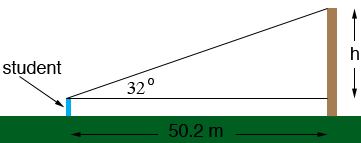SEARCH HOMEMath Central Quandaries & QueriesQuestion from lalaine, a student: Hi, this is my problem.. From a point 50.2 m to the pole, a student measured the angle of elevation to the top of the pole to be 32°. Find the height of the pole if the student's height from his feet to his eyes is about 4 ft.Lalaine,

I drew a sketch of what you described.The height of the pole is h plus the height of the student from his feet to his eyes. This height of the student is 4 feet which is 1.22 m thus the height of the pole is h + 1.22 m.

The right triangle in the diagram has an angle with measure 32o. The side adjacent to this angle has length 50.2 m and the opposite this angle has length h m. What trig function relates the angle, the opposite side and the adjacent side?

PennyMath Central is supported by the University of Regina and The Pacific Institute for the Mathematical Sciences.